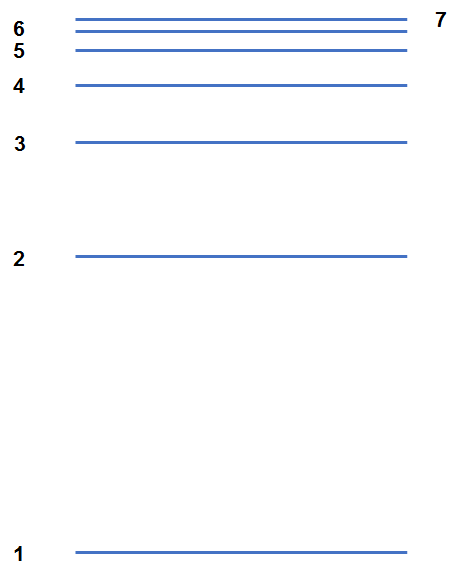# Problem: Calculate the longest and shortest wavelengths of light emitted by electrons in the hydrogen atom that begin in the n = 6 state and then fall to states with smaller values of  n.

###### FREE Expert SolutionDistance = Energy

lower-numbered shell there’s a bigger distance between them
the distance between shells gets smaller the higher up you go
the smaller the distance then the less energy is required for an electron to travel

$\overline{){\mathbf{E}}{\mathbf{=}}\frac{\mathbf{hc}}{\mathbf{\lambda }}}$

↓ smaller distance, ↓ less energy required, ↑ wavelength

longest wavelength:     n = 6 to n = 5

↑ bigger distance, ↑ higher energy required,  wavelength

shortest wavelength:     n = 6 to n = 1

We’re going to use the Balmer Equation which relates wavelengths to a photon’s electronic transitions.

$\overline{)\frac{\mathbf{1}}{\mathbf{\lambda }}{\mathbf{=}}{{\mathbf{RZ}}}^{{\mathbf{2}}}\left(\frac{\mathbf{1}}{{{\mathbf{n}}^{\mathbf{2}}}_{\mathbf{final}}}\mathbf{-}\frac{\mathbf{1}}{{{\mathbf{n}}^{\mathbf{2}}}_{\mathbf{initial}}}\right)}$

λ = wavelength, m
R = Rydberg constant = 1.097x107 m-1
Z = atomic number of the element
ninitial = initial energy level
nfinal = final energy level

Calculate the wavelength of light (λ):

n = 6 to n = 5

Z = atomic number of Hydrogen = 1 (refer to the periodic table)
R = 1.097x107 m
-1
ninitial = 1
nfinal = 3

λ = 7458 nm

n = 6 to n = 1

94% (36 ratings)###### Problem Details

Calculate the longest and shortest wavelengths of light emitted by electrons in the hydrogen atom that begin in the n = 6 state and then fall to states with smaller values of  n.

Frequently Asked Questions

What scientific concept do you need to know in order to solve this problem?

Our tutors have indicated that to solve this problem you will need to apply the Bohr Equation concept. You can view video lessons to learn Bohr Equation. Or if you need more Bohr Equation practice, you can also practice Bohr Equation practice problems.

What professor is this problem relevant for?

Based on our data, we think this problem is relevant for Professor McCrory's class at UMICH.

What textbook is this problem found in?

Our data indicates that this problem or a close variation was asked in Chemistry: An Atoms First Approach - Zumdahl Atoms 1st 2nd Edition. You can also practice Chemistry: An Atoms First Approach - Zumdahl Atoms 1st 2nd Edition practice problems.